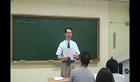### 주메뉴

### 원자력공학개론

• 포항공과대학교
• John C. Lee• 주제분류
공학 >정밀ㆍ에너지 >원자력공학
• 강의학기
2011년 1학기
• 조회수
8,336
•
This course will cover an introduction to nuclear power plants, interaction of radiation with matter, neutron cross sections, diffusion theory, criticality calculations, reactor kinetics, neutron slowing down theory, heat transport and temperature distribution in reactor core, and reactivity feedback.
Introduction to Nuclear Power Plants#### 차시별 강의1Introduction to Nuclear Power Plants Introducing fundamentals of nuclear reactor phyiscs & engineering (4.26)4Two-body collision mechanics Inducing the relations between Lab frame & CM (5.2)6Differential cross section, neutron number density Explainations on differential, integral cross section, and scattering krnel (5.6)7Angular neutron flux, scalar flux, net current, partial current Interpreting definitions of neutron flux and current (5.9)8Reaction rate, effective cross sections Interpreting means of reaction rate & effective cross section (5.11)9Numerical integration for Prob. 2.1, 4-factor formula, diffusion equation 1. Inducing the flux for neutrons obeying the Maxwell-Boltxman distribution 2. Introducing the neutron diffusion equation. (5.13)11Diffusion coefficient, mean cosine scattering angle, 1-group diff. equation 1. providing an interpretation of the diffusion coefficient for the current. 2. Discussing limitations and applicability of diffusion theory. 3. Derivation of the one-group diffusion equation.(5.18)12Boundary conditions for diffusion equation, plane source problem 1. disscusion of the boundary conditions necessary for the solution of Eq. 4.1. 2. providing source conditons for solutions of the steady-state diffusion equation (5.20)13Review on neutron flux, mean square distance, finite slab problem providing solutions of the steady-state diffusion equation according to each source type (5.23)14Neutron albedo providing the solution of the steady-state diffusion equation incase of two slabs of finite thickness (5.3)15P.S. #3, distributed source problem, kernel technique providing the solution fo the diffusion equation with distributed sources (6.1)16P.S. #3, multiplying medium, geometric and material buckling introducing the concept of material and geometric bucklings together with the one-group form of the effective mutiplication factor (6.3)#### 연관 자료#### 사용자 의견

강의 평가를 위해서는 로그인 해주세요.

#### 이용방법

• 강의 이용시 필요한 프로그램 [바로가기]

※ 강의별로 교수님의 사정에 따라 전체 차시 중 일부 차시만 공개되는 경우가 있으니 양해 부탁드립니다.